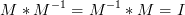Matrix tutorial¶

Project owner: Eugene Lazutkin

Linear transformations are very important part of any graphics library. We deal with 2D graphics, it means we operate with 3 by 3 matrices: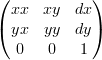Because the third row is always constant we use an abbreviated way to write it: {xx: 1, xy: 0, yx: 0, yy: 1, dx: 0, dy: 0} — this is an identity matrix.

The same simplification goes for coordinates: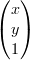Because the third element is always 1 we “add” it virtually: {x: 12, y: 33}.

The way to apply a matrix to a coordinate (to multiply a coordinate by a matrix) u = M * v — where, v is an input vector (e.g., {x: 1, y: 2}), M is a transformation matrix, u is the resulting vector, and * denotes the multiplication operation: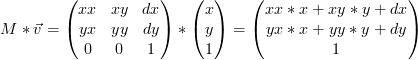The matrix multiplication can be written like this (* is omitted in this equation like in regular math to conserve space):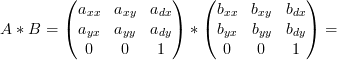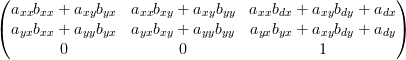(In all other examples we will skip the dummy third row because it is always the same).

The way to combine transformations together:

A * B * C * p == (A * B) * C * p == A * (B * C) * p == A * B * (C * p) == (A * B * C) * p, and so on, where A, B, and C are transformation matrices, p is a coordinate vector, and * is a multiplication operation. The result of all these calculations is the same final coordinate. Basically it means that you can combine them in any way you like as long as the relative order is preserved.

This is the very important fact that gives us the ability to combine transformations together to improve the performance and to simplify algorithms.

Effectively all transformations are always applied from right to left sequentially, and they can be combined producing a matrix, which defines a complex transformation.

dojox.gfx defines several constants and functions to deal with matrices. Below is the list of their formal definitions:

• identity does nothing: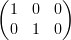• flipX reflects a point around the Y axis by changing a sign of all X components: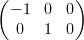• flipY reflects a point around the X axis by changing a sign of all Y components: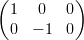• flipXY reflects a point around the beginning of coordinates (0, 0) by changing a sign of both components: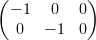• translate(dx, dy) moves a point horizontally by shifting it dx units, and vertically by shifting it dy units: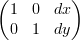• scale(sx, sy) scales a picture by sx factor horizontally and sy factor vertically:• rotate(r) rotates a point around (0, 0) by r radians: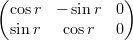• skewX(r) skews a picture in the X dimension by r radians: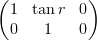• skewY(r) skews a picture in the Y dimension by r radians: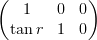• reflect(x, y) reflects a point around the vector from (0, 0) to (x, y):

let a=x, b=y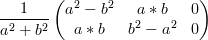• project(x, y) projects a point picture orthogonally on the vector from (0, 0) to (x, y):

let a=x, b=y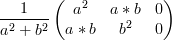• invert(M) creates an inverted matrix to “undo” the parameter M: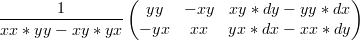Inverse matrices have a very useful property (where M is a matrix, I is an identity matrix):